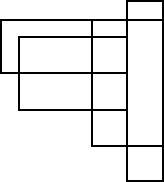# How many four-sided figures appear in the diagram?

There are a number of these geometric combinometrics problems around.  Here is a complete graphic solution to the one of the more common ones.

Question: How many four-sided figures appear in the diagram below?

1. 10
2. 16
3. 22
4. 25
5. 28